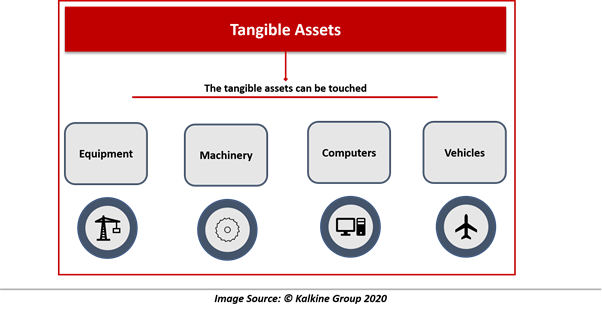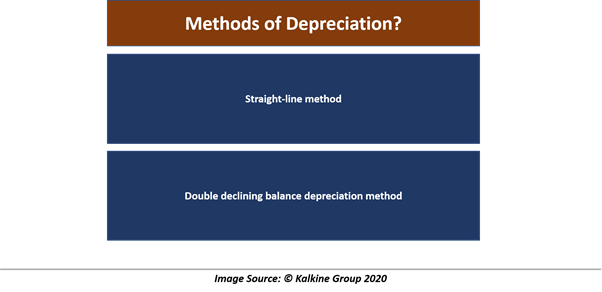# Half-Year Convention for Depreciation

• Updated on

## What is depreciation?

Depreciation is an accounting method used to allocate the cost of a tangible asset to the books of accounts over the useful life of the asset. It is essentially the accounting for wear and tear on the asset over its useful life.

Depreciation also refers to the value of the asset that has been used over time. Assets of a firm that are used for over a one-year period largely include physical assets. Although firms incur expense while purchasing these assets, the expenses are not charged in the income statement.

Such assets are recorded in the balance sheet of the firm and are expensed on the income statement as depreciation expense over time during the life of the asset. The tax authorities also decide the useful life of assets because overstating depreciation expense can lower tax liability.

Now assets come in two variety: tangible assets and intangible assets. As the name suggests tangible, the tangible assets can be touched, such as equipment, machinery, computers, vehicles etc. Depreciation is used to expense the tangible assets of a firm.Intangible assets cannot be touched and include assets like licenses, copyrights, patents, brand names, logos etc. Amortisation of assets is an accounting method similar to depreciation used to expense intangible assets.

Long-term assets are the source of generating revenue for firms over a long period of time, therefore the cost of acquiring tangible long-term assets is not expensed fully at the time of purchases and is expensed over the life of the asset.

As the asset is used over periods, the carrying value of an asset in the balance sheet is reduced over time. Carrying value of an asset is the original cost minus accumulated depreciation on the asset over time.

Since the cost of acquiring the long-term tangible asset is not expensed fully at the time of purchase and is expensed over its useful life, the depreciation expense is a non-cash charge because actual cash outgo was incurred at the time of purchase.

But depreciation expense reduces the reported earnings of the company as it is charged on the income statement of the firm. Since the expenses are deducted from the revenue of the firm, the tax liability of the firm is also reduced.

## What are the methods of depreciation?## Straight-line method

The straight-line method is the most common method of depreciating an asset over its life. Under this method, the recurring depreciating amount of the asset remains constant and is not changed over the life of the asset.

For example, a firm buys a machine for \$10000 with a salvage value of \$2000, and the useful life of the asset is ten years. The depreciable value of the asset will be \$8000, which is the cost of machine minus salvage value.

Now the firm will depreciate the \$8000 each year at a rate of \$800 per year. The per-year depreciation charge of \$800 is the depreciable value of the asset divided by the useful life of the asset (8000/10).

## Double declining balance depreciation method

It is an accelerated type of depreciation method. Under this method, the depreciation expense in higher in the beginning years and gradually reduces over the life of an asset. It also reflects that assets are more valuable in the early years of production compared to later years.

In this method, the subsequent depreciation charges after the initial charge are calculated using the ending balance of the asset in the last period. Ending balance of the asset is the original cost of the asset less accumulated depreciation. Also, the depreciation factor in this method is twice of the straight-line method.

Depreciation expense = (100%/Useful life of asset) x 2

## Why is depreciation due diligence important?

Depreciation can be used to manipulate the financials of the company. Overstating and understating depreciation charges directly impacts the profit of the company. When a firm is charging less depreciation than required, it would directly increase the profits of the firm.

When depreciation expense is lesser than the actual expense, the income statement will record lower amount of expenses, therefore the deductions from revenue will lesser and profits will increase.

Investors also assess whether the useful life of asset used in calculating the depreciation of firm is appropriate or not. The companies should use an appropriate useful life of the asset. When the useful life of the asset is increased, the depreciation charges will spread across an increased number of years.

As a result, the depreciation expenses during the life of an asset would be understated since the actual life of an asset is less than recorded. Investors prefer checking the number of years used as the useful life of an asset.

Sometimes firms may choose to change the method of depreciation. Although it could be appropriate when actual business conditions don’t match the method adopted, there remains a possibility that the decision to change the method could be driven by the motive to manipulate depreciation expenses.

Companies may seek to keep the assets in the balance sheet even though the asset is of no use. This will help the company to keep incurring depreciation expense on the income statement and reduce the tax liability of the business.

When the value of assets of the company has appreciated in light of the market environment, the balance sheet value of the asset will also increase. When the balance sheet value of an asset is increased, the depreciation charges should also increase. Therefore, appreciation in the value of an asset should also increase depreciation expense for the company.

##### Top ASX Listed Companies

Definition:
We use cookies to ensure that we give you the best experience on our website. If you continue to use this site we will assume that you are happy with it. OK